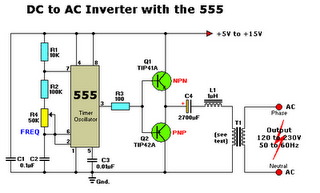## Jumat, Maret 18, 2011

### DC to AC inverter with the 555Series of DC-AC inverter produces AC output voltage as voltage net. IC 555 configured as a low frequency oscillator. Frequency can be changed from 50 to 60 Hz with a frequency potensio meter change R4.

This DC-to-AC inverter schematic produces an AC output at line frequency and voltage. The 555 is configured as a low-frequency oscillator, tunable over the frequency range of 50 to 60 Hz by Frequency potentiometer R4.

The 555 feeds its output (amplified by Q1 and Q2) to the input of transformer T1, a reverse-connected filament transformer with the necessary step-up turns ratio. Capacitor C4 and coil L1 filter the input to T1, assuring that it is effectively a sine wave. Adjust the value of T1 to your voltage.

The output (in watts) is up to you by selecting different components.

Input voltage is anywhere from +5V to +15Volt DC, adjust the 2700uF cap's working voltage accordingly.
Replacement types for Q1 are: TIP41B, TIP41C, NTE196, ECG196, etc. Replacement types for Q2 are: TIP42B, TIP42C, NTE197, ECG197, etc. Don't be afraid to use another type of similar specs, it's only a transistor... ;-)

Parts List:

R1 = 10K
R2 = 100K
R3 = 100 ohm
R4 = 50K potmeter, Linear
C1,C2 = 0.1uF
C3 = 0.01uF
C4 = 2700uF
Q1 = TIP41A, NPN, or equivalent
Q2 = TIP42A, PNP, or equivalent
L1 = 1uH
T1 = Filament transformer, your choice

If the whole thing is working, good. If not, relax and don't get frustrated. Do the following checks:

1. You have connected the filament transformer in REVERSE yes?
2. If not, disconnect the power and reverse. If you have, disconnect the transformer and measure the voltage after L1 and ground.
3. Just in case, GROUND for this circuit is same as negative (-).
4. Q1/Q2 are oposites, e.i. npn/pnp.
5. Is your 555 perhaps defective? Disconnect R3 from pin 3 and check pin 3 for a pulse.
6. Check your transistors to make sure they are not defective.

Error fix: Pin 7 and 2 were reversed. Original pinout was correct.